# sklearn.cluster.KMeans¶

class sklearn.cluster.KMeans(n_clusters=8, init='k-means++', n_init=10, max_iter=300, tol=0.0001, precompute_distances='auto', verbose=0, random_state=None, copy_x=True, n_jobs=None, algorithm='auto')[source]

K-Means clustering

Read more in the User Guide.

Parameters: n_clusters : int, optional, default: 8 The number of clusters to form as well as the number of centroids to generate. init : {‘k-means++’, ‘random’ or an ndarray} Method for initialization, defaults to ‘k-means++’: ‘k-means++’ : selects initial cluster centers for k-mean clustering in a smart way to speed up convergence. See section Notes in k_init for more details. ‘random’: choose k observations (rows) at random from data for the initial centroids. If an ndarray is passed, it should be of shape (n_clusters, n_features) and gives the initial centers. n_init : int, default: 10 Number of time the k-means algorithm will be run with different centroid seeds. The final results will be the best output of n_init consecutive runs in terms of inertia. max_iter : int, default: 300 Maximum number of iterations of the k-means algorithm for a single run. tol : float, default: 1e-4 Relative tolerance with regards to inertia to declare convergence precompute_distances : {‘auto’, True, False} Precompute distances (faster but takes more memory). ‘auto’ : do not precompute distances if n_samples * n_clusters > 12 million. This corresponds to about 100MB overhead per job using double precision. True : always precompute distances False : never precompute distances verbose : int, default 0 Verbosity mode. random_state : int, RandomState instance or None (default) Determines random number generation for centroid initialization. Use an int to make the randomness deterministic. See Glossary. copy_x : boolean, optional When pre-computing distances it is more numerically accurate to center the data first. If copy_x is True (default), then the original data is not modified, ensuring X is C-contiguous. If False, the original data is modified, and put back before the function returns, but small numerical differences may be introduced by subtracting and then adding the data mean, in this case it will also not ensure that data is C-contiguous which may cause a significant slowdown. n_jobs : int or None, optional (default=None) The number of jobs to use for the computation. This works by computing each of the n_init runs in parallel. None means 1 unless in a joblib.parallel_backend context. -1 means using all processors. See Glossary for more details. algorithm : “auto”, “full” or “elkan”, default=”auto” K-means algorithm to use. The classical EM-style algorithm is “full”. The “elkan” variation is more efficient by using the triangle inequality, but currently doesn’t support sparse data. “auto” chooses “elkan” for dense data and “full” for sparse data. cluster_centers_ : array, [n_clusters, n_features] Coordinates of cluster centers. If the algorithm stops before fully converging (see tol and max_iter), these will not be consistent with labels_. labels_ : Labels of each point inertia_ : float Sum of squared distances of samples to their closest cluster center. n_iter_ : int Number of iterations run.

MiniBatchKMeans
Alternative online implementation that does incremental updates of the centers positions using mini-batches. For large scale learning (say n_samples > 10k) MiniBatchKMeans is probably much faster than the default batch implementation.

Notes

The k-means problem is solved using either Lloyd’s or Elkan’s algorithm.

The average complexity is given by O(k n T), were n is the number of samples and T is the number of iteration.

The worst case complexity is given by O(n^(k+2/p)) with n = n_samples, p = n_features. (D. Arthur and S. Vassilvitskii, ‘How slow is the k-means method?’ SoCG2006)

In practice, the k-means algorithm is very fast (one of the fastest clustering algorithms available), but it falls in local minima. That’s why it can be useful to restart it several times.

If the algorithm stops before fully converging (because of tol or max_iter), labels_ and cluster_centers_ will not be consistent, i.e. the cluster_centers_ will not be the means of the points in each cluster. Also, the estimator will reassign labels_ after the last iteration to make labels_ consistent with predict on the training set.

Examples

>>> from sklearn.cluster import KMeans
>>> import numpy as np
>>> X = np.array([[1, 2], [1, 4], [1, 0],
...               [10, 2], [10, 4], [10, 0]])
>>> kmeans = KMeans(n_clusters=2, random_state=0).fit(X)
>>> kmeans.labels_
array([1, 1, 1, 0, 0, 0], dtype=int32)
>>> kmeans.predict([[0, 0], [12, 3]])
array([1, 0], dtype=int32)
>>> kmeans.cluster_centers_
array([[10.,  2.],
[ 1.,  2.]])


Methods

 fit(X[, y, sample_weight]) Compute k-means clustering. fit_predict(X[, y, sample_weight]) Compute cluster centers and predict cluster index for each sample. fit_transform(X[, y, sample_weight]) Compute clustering and transform X to cluster-distance space. get_params([deep]) Get parameters for this estimator. predict(X[, sample_weight]) Predict the closest cluster each sample in X belongs to. score(X[, y, sample_weight]) Opposite of the value of X on the K-means objective. set_params(**params) Set the parameters of this estimator. transform(X) Transform X to a cluster-distance space.
__init__(n_clusters=8, init='k-means++', n_init=10, max_iter=300, tol=0.0001, precompute_distances='auto', verbose=0, random_state=None, copy_x=True, n_jobs=None, algorithm='auto')[source]
fit(X, y=None, sample_weight=None)[source]

Compute k-means clustering.

Parameters: X : array-like or sparse matrix, shape=(n_samples, n_features) Training instances to cluster. It must be noted that the data will be converted to C ordering, which will cause a memory copy if the given data is not C-contiguous. y : Ignored not used, present here for API consistency by convention. sample_weight : array-like, shape (n_samples,), optional The weights for each observation in X. If None, all observations are assigned equal weight (default: None)
fit_predict(X, y=None, sample_weight=None)[source]

Compute cluster centers and predict cluster index for each sample.

Convenience method; equivalent to calling fit(X) followed by predict(X).

Parameters: X : {array-like, sparse matrix}, shape = [n_samples, n_features] New data to transform. y : Ignored not used, present here for API consistency by convention. sample_weight : array-like, shape (n_samples,), optional The weights for each observation in X. If None, all observations are assigned equal weight (default: None) labels : array, shape [n_samples,] Index of the cluster each sample belongs to.
fit_transform(X, y=None, sample_weight=None)[source]

Compute clustering and transform X to cluster-distance space.

Equivalent to fit(X).transform(X), but more efficiently implemented.

Parameters: X : {array-like, sparse matrix}, shape = [n_samples, n_features] New data to transform. y : Ignored not used, present here for API consistency by convention. sample_weight : array-like, shape (n_samples,), optional The weights for each observation in X. If None, all observations are assigned equal weight (default: None) X_new : array, shape [n_samples, k] X transformed in the new space.
get_params(deep=True)[source]

Get parameters for this estimator.

Parameters: deep : boolean, optional If True, will return the parameters for this estimator and contained subobjects that are estimators. params : mapping of string to any Parameter names mapped to their values.
predict(X, sample_weight=None)[source]

Predict the closest cluster each sample in X belongs to.

In the vector quantization literature, cluster_centers_ is called the code book and each value returned by predict is the index of the closest code in the code book.

Parameters: X : {array-like, sparse matrix}, shape = [n_samples, n_features] New data to predict. sample_weight : array-like, shape (n_samples,), optional The weights for each observation in X. If None, all observations are assigned equal weight (default: None) labels : array, shape [n_samples,] Index of the cluster each sample belongs to.
score(X, y=None, sample_weight=None)[source]

Opposite of the value of X on the K-means objective.

Parameters: X : {array-like, sparse matrix}, shape = [n_samples, n_features] New data. y : Ignored not used, present here for API consistency by convention. sample_weight : array-like, shape (n_samples,), optional The weights for each observation in X. If None, all observations are assigned equal weight (default: None) score : float Opposite of the value of X on the K-means objective.
set_params(**params)[source]

Set the parameters of this estimator.

The method works on simple estimators as well as on nested objects (such as pipelines). The latter have parameters of the form <component>__<parameter> so that it’s possible to update each component of a nested object.

Returns: self
transform(X)[source]

Transform X to a cluster-distance space.

In the new space, each dimension is the distance to the cluster centers. Note that even if X is sparse, the array returned by transform will typically be dense.

Parameters: X : {array-like, sparse matrix}, shape = [n_samples, n_features] New data to transform. X_new : array, shape [n_samples, k] X transformed in the new space.

## Examples using sklearn.cluster.KMeans¶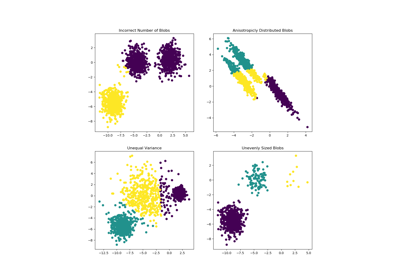Demonstration of k-means assumptions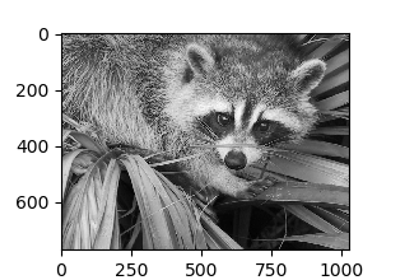Vector Quantization Example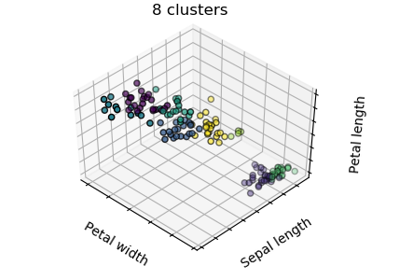K-means ClusteringColor Quantization using K-Means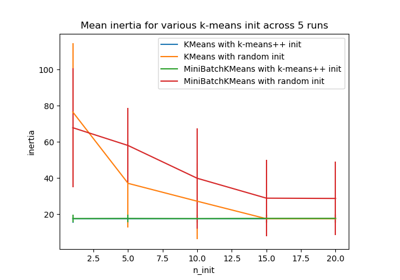Empirical evaluation of the impact of k-means initialization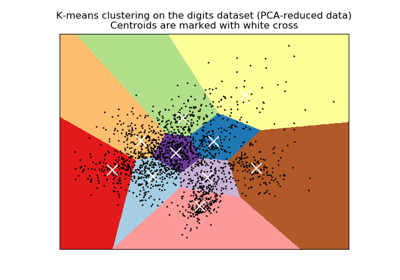A demo of K-Means clustering on the handwritten digits data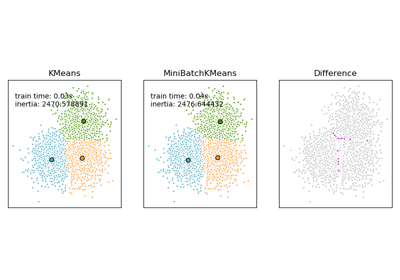Comparison of the K-Means and MiniBatchKMeans clustering algorithms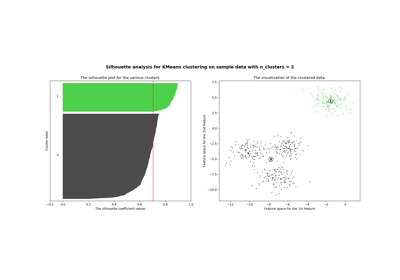Selecting the number of clusters with silhouette analysis on KMeans clusteringClustering text documents using k-means